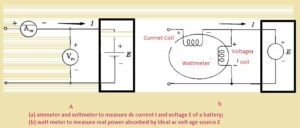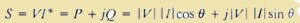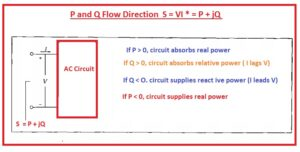Hello, friends welcome to a new post in this post we will have a detailed look at Direction Of Power Flow In Ac System. There is main power system in the ac is the alternating voltage that is delivering to load on regular basis after the generation system. This system consists of three types of main power active, reactive, and apparent.

In this post, we cover the details of power flow and its direction in the system. So let get started

Direction Of Power Flow In Ac System

• To study the basics of power flow direction in the system the main parameters that are taken into consideration are active power, apparent power, and voltage generation and their relation among them.
• Here the main point to be noted is that power is either generating or using through the system through discussing the power flow direction when voltage and current have certain values.
• This point is also applicable for dc system whether power is generating and using through the system.
• In below figure you can see the circuitry where current and voltage are passing• In circuitry, if the value of Voltage is one hundred volts and current is ten amperes so the power delivered the battery will be multiple of both current and voltage and its 1000watts
• If now we reverse the connection of the ammeter then results will be the same but with different polarity. -1000Watt is the answer. It means the battey is gettig discahrge
• In figure denoted as b ac system is shown have ideal voltage source that has positive polarity for instantaneous voltage.
• current is flowing in the box for the positive half cycle.
• In figure denoted b there is a wattmeter that has a current coil and voltage coil in circuitry.
• For measures of power both the coils should be attached in proper way to the circuitry
• As the power used by the circuitry is given here.• In the above equation θ angle through which current is lagging volts.
• If there i upscale at the wattmeter according to connection shown in circuitry denoted above with b the term for above equation P=VIcosθ has positive value and real power is used through system
• If there is a downscale int the meter then  P=VIcosθ has a negative value  and by changing one connection either current or potential coil we come to see that power having positive value is delivering though system• If the wattmere is cahnted to varmter these resutsl also work work reativ power.
• In a simple way, we can find the value of reactive and active power by using the above circuitry. through using the value of polarity for current and voltage according to below shown
• the vale of real and imaginary portion of the equation S = VI* can be found through the use of power and Q given by the circuitry
• If the other is currently lagging the voltage through angle lies between zero and ninety  then  P=VIcosθ and  P=VIsinθ has a positive value